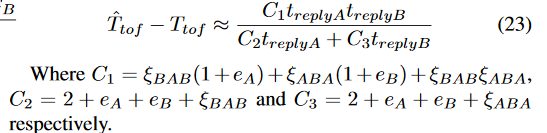# SDS TWR Sample Codes

Hi,

I’m using EVK1000 and disconnected the onboard arm and connected it to my own controller. Currently, im using the Asymmetric TWR Formula as shown below.

But it has large deviation like the values are incrementing up to 100cm for an 8m ranging. So I’m suspecting that there is some clock/frequency drift between the two device. Hence, I’m trying new approach on the SDS TWR Formula as shown below.Do anyone have sample codes on the similar approach? Is it possible for me to refer to it?

Thank you so much!
-Kevin

The first formula should work fine.

The decawave clocks work out as giving around 4.5mm resolution. If you are seeing ~100 cm quantization in the results that is around 220 times worse than expected.

Any chance you are using 32 bit integer maths (or even worse floating point) rather than 64 bit integers when doing calculations on the timestamps? Once you have the time differences you can normally use 32 bits but for those initial time period calculations you need more. The registers are 40 bits, if you don’t use a 64 bit int you’ll get rounding errors.

Hi Andy,

I have tried to change the data type to calculate the Ra, Rb, Da, Db to 64 bit unsigned integer and I got this output. Is there any cause for this kind of output(centimetres)? When the value is correct, it still does drift.

For your reference on the 100cm drifts as I mention previously.

This is the code I use that works well for me:

First wrap around is checked for all time periods:

``````localRangeTime = RxTimestamp;
if (localRangeTime < TxTimestamp
localRangeTime -= TxTimestamp;
``````

And then assuming all times are valid:

``````//This is done to retain the resolution after averaging out all the times
uint64_t rrHD = remoteRangeTime<<2;
uint64_t lrHD = localRangeTime<<2;
uint64_t reHD = firstEchoDelay<<2;
uint64_t leHD = secondEchoDelay<<2;
uint64_t tprop = (rrHD*lrHD - reHD*leHD) / (rrHD + lrHD + reHD + leHD);

float distance = tprop * c_mPerTick/4; // divide by 4 to cancel bit shift effects.
``````

The bit shifting is so that we can keep things as integers for as long as possible and still avoid any rounding issues. But this is only going to make a 1-2 cm difference at most.

I can’t see why you’re getting the results you do but I don’t think the issue is your range calculation code.